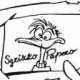# (pseudo-/quasi-)convexitiy of ratio between quadratic and affine function

 0 Let $$X\subseteq\mathbb{R}^n$$. I have the following function $$f:X\rightarrow\mathbb{R}$$: $$f({\bf x})= \frac{c_1 + \sum_{i=1}^n a_ix_i +\sum_{i=1}^n b_ix_i^2}{c_2+\sum_{i=1}^n d_i x_i}\enspace.$$ All the $$a_i$$, $$b_i$$, $$c_i$$, and $$d_i$$ are strictly greater than 0, and X is such that $$c_2+\sum_{i=1}^n d_i x_i>0, \forall {\bf x}\in X\enspace.$$ Is $$f$$ convex or at least pseudo- or quasi-convex? Note that $$f({\bf x})= \frac{c_1 + \sum_{i=1}^n a_ix_i }{c_2+\sum_{i=1}^n d_i x_i}+\frac{\sum_{i=1}^n b_ix_i^2}{c_2+\sum_{i=1}^n d_i x_i}$$ and the first term is on the right side is pseudo-linear (hence pseudo-convex, hence quasi-convex) and third term is convex (hence pseudo-convex, hence quasi-convex). I know that the sum of quasi-convex functions is not in general quasi-convex, but I wonder whether something else was known that can help me show that $$f$$ is. asked 28 Oct '13, 18:29MatteoR 1●2 accept rate: 0%Paul Rubin ♦♦ 14.6k●5●13 Never mind: my original function was actually $\frac{\sum_{i=1}^\ell (b_i + \sum_{j=1}^\ell a_j^{(i)} x_j)^2}{c_1+\sum_{i=1}^n d_ix_i}$ so I can see it as a sum of $$g_i^2/h$$, where $$g_i$$ is affine (and actually non-negative in my domain) and $$h$$ is positive affine. Then each of the $$g_i^2/h$$ is convex, and so is their sum. (29 Oct '13, 08:57) MatteoR

 1 In fact your constraint belongs to the class cf conic quadratic (aka. SOCP) representable sets that is known to be convex. Also problems with conic quadratic representable sets can be solved efficiently. It is easy to your constraint can be represented as follows $$\left[ \begin{array}{c} 0.5 \\ dx+c \\ Ax+b \end{array} \right] \in K$$ where $$K := \{y \mid 2y_1 y_2 \geq \|y_{3:n}\|^2, \quad y_1,y_2 \geq 0\}.$$ K is the so called rotated quadratic cone. For more information see the MOSEK modelling manual. answered 31 Oct '13, 03:49Erling_MOSEK 616●1●4 accept rate: 3%fbahr ♦ 4.6k●7●16 I have tested the LaTex code on mathjax.org where shows correct but here are problems. Do not know why. (31 Oct '13, 03:52) Erling_MOSEK @Erling: OR-X FAQ: Hey, how do I get that fancy math stuff? $$\rightarrow$$ "Within latex, all backslashes must be doubled." (31 Oct '13, 05:36) fbahr ♦ Thanks a lot Florian. (31 Oct '13, 06:19) Erling_MOSEK
 toggle preview community wiki

By Email:

Markdown Basics

• *italic* or _italic_
• **bold** or __bold__
• image?![alt text](/path/img.jpg "Title")
• numbered list: 1. Foo 2. Bar
• to add a line break simply add two spaces to where you would like the new line to be.
• basic HTML tags are also supported

Tags: